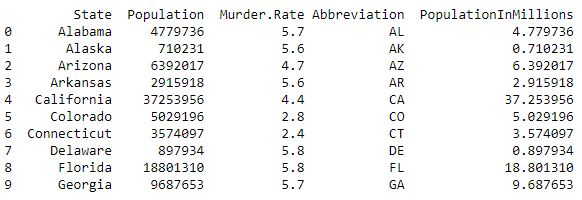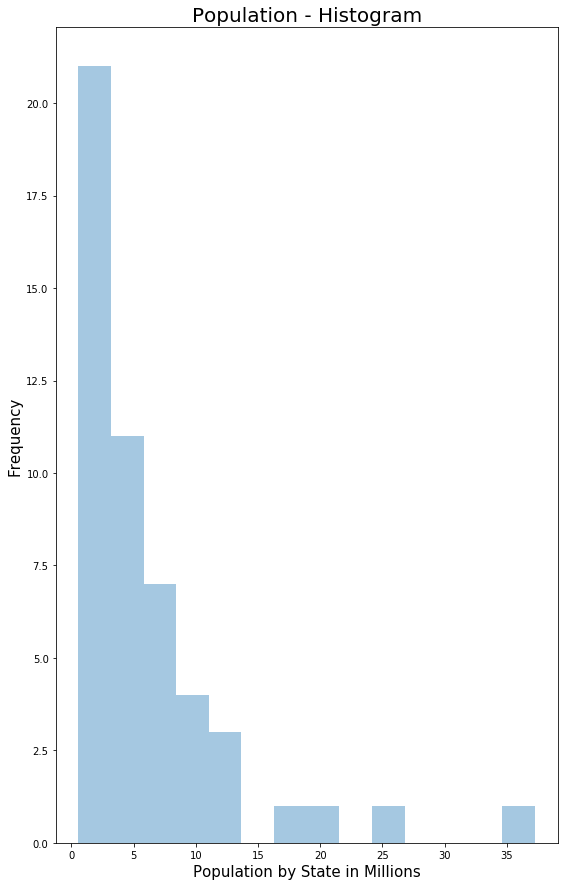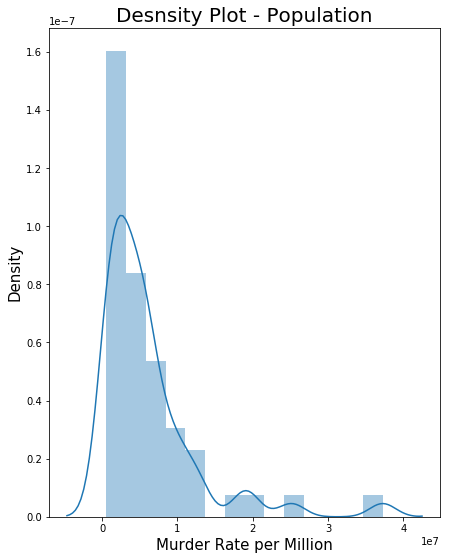GeeksforGeeks App
Open AppBrowser
Continue

# Exploring Data Distribution | Set 2

Prerequisite: Exploring Data Distribution | Set 1
Terms related to Exploration of Data Distribution

```-> Boxplot
-> Frequency Table
-> Histogram
-> Density Plot```

## Python3

 `import` `numpy as np``import` `pandas as pd``import` `seaborn as sns``import` `matplotlib.pyplot as plt`

## Python3

 `data ``=` `pd.read_csv(``"../data/state.csv"``)` `# Adding a new column with derived data``data[``'PopulationInMillions'``] ``=` `data[``'Population'``]``/``1000000` `print` `(data.head(``10``))`

Output :• Histogram: It is a way of visualizing data distribution through frequency table with bins on the x-axis and data count on the y-axis.
Code – Histogram

## Python3

 `# Histogram Population In Millions` `fig, ax2 ``=` `plt.subplots()``fig.set_size_inches(``9``,  ``15``)` `ax2 ``=` `sns.distplot(data.PopulationInMillions, kde ``=` `False``)``ax2.set_ylabel(``"Frequency"``, fontsize ``=` `15``)``ax2.set_xlabel(``"Population by State in Millions"``, fontsize ``=` `15``)``ax2.set_title(``"Population - Histogram"``, fontsize ``=` `20``)`

• Output :• Density Plot: It is related to histogram as it shows data-values being distributed as continuous line. It is a smoothed histogram version. The output below is the density plot superposed over histogram.
Code – Density Plot for the data

## Python3

 `# Density Plot - Population` `fig, ax3 ``=` `plt.subplots()``fig.set_size_inches(``7``,  ``9``)` `ax3 ``=` `sns.distplot(data.Population, kde ``=` `True``)``ax3.set_ylabel(``"Density"``, fontsize ``=` `15``)``ax3.set_xlabel(``"Murder Rate per Million"``, fontsize ``=` `15``)``ax3.set_title(``"Density Plot - Population"``, fontsize ``=` `20``)`

• Output :My Personal Notes arrow_drop_up